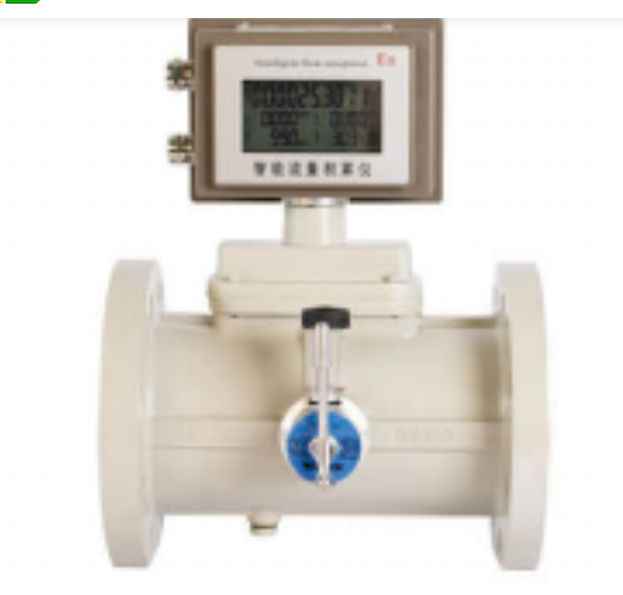Home   |   INFO CENTER   |   NEWS   |

# Introduction of flow measurement

2022-11-28

The ability to measure flow rate is another important process measurement. There are different classes of flow measurement transducers: differential pressure measurement, velocity-based measurement, positive displacement measurement, and mass flow measurement.

Differential pressure measurement devices can be separated into restrictive and non-restrictive devices. When fluid flows through a restrictive flow element, there is a differential pressure across that element. This pressure is directly related to volume flow rate. Since we are measuring a differential pressure, most any of the above pressure transducers may be used to detect that pressure.

In each case, the pressure transmitted is a function of the fluid velocity. Of course, if one knows fluid velocity, one may apply the continuity equation to determine the volume flow rate. The problem with the pitot-static tube is it only measures velocity at one point, usually the center line. However, since the velocity of flow is variable along the cross-section with a maximum occurring at the center line, the resulting signal only indicates center line velocity, not average velocity. In theory, we could develop a relationship between this maximum and center line velocity, but this tends to be difficult to do in practice.Operating on the same principle as the pitot-static tube, the annubar detects the average velocity pressure across the cross-section of the duct or pipe.

Vortex Shedding Flow Meter

When fluid flows over an object, the flow separates from the object at certain points. If the object is properly shaped, the way the vortex flow meter accuracy separates is predictable. As the flow separates, a vortex is formed. This vortex exerts a pressure different from the local static pressure and proportional to fluid flow velocity.Turbine Type Flow Meter

Velocity based customized gas turbine flow meter detection is accomplished with a turbine or paddlewheel sensor, a vortex shedding sensor, a magnetic sensor, or an ultrasonic sensor. The turbine/paddlewheel sensor places a rotating element into the flow stream. As the element rotates, it rotates by a magnetic pickup resulting in a very low voltage pulse. A transmitter converts this pulse into a standard process signal.

Magnetic flowmeter

A magnetic flow meter works on the basis of fundamental electrical and magnetic laws. In such a meter, the fluid acts as the conductor. The meter itself is constructed of a nonconductive material. Surrounding the meter is a series of electrical coils and sensor pickups. The electrical coils are used to generate a magnetic field. As the fluid moves through the magnetic field, a voltage is induced in the fluid. The sensors detect the magnitude of this voltage, which is proportional to the velocity of the fluid flow.

Chat Online 编辑模式下无法使用